# Picturing the Trigonometric Ratios: Step-by-step

Let’s start with the unit circle and a central angle θ that meets an arc on the circle: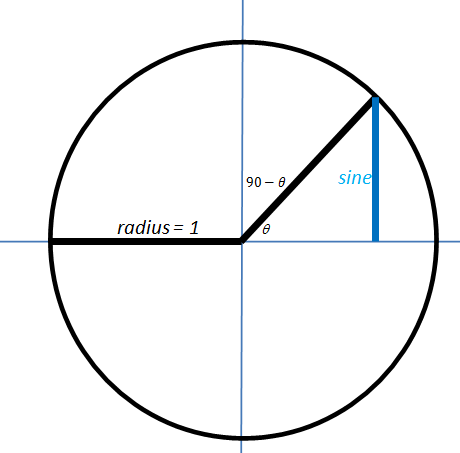The blue segment is called the “sine” of the angle.  It gets its name from a word that means “bowstring”.  Here is a diagram of a bow string truss.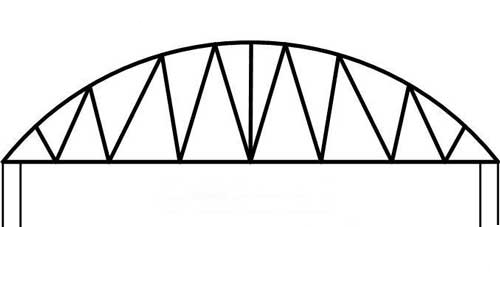So if you think of the intercepted arc as the bow and focus only on the upper half…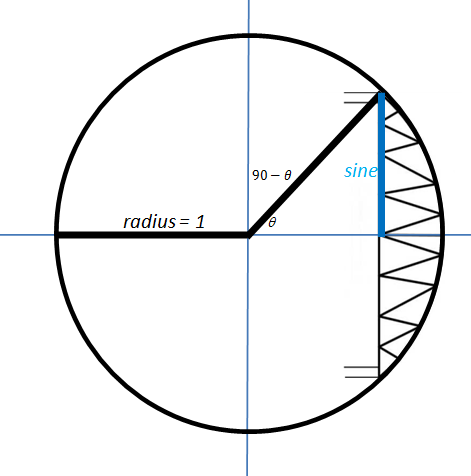… you can see how they first decided to call that segment the “bowstring.”

But you do not have to hold your bow vertically.  If you use the complementary angle, 90-θ, you get the horizontal segment I have drawn in red (and re-drawn on the x-axis as well…you’ll see why soon.)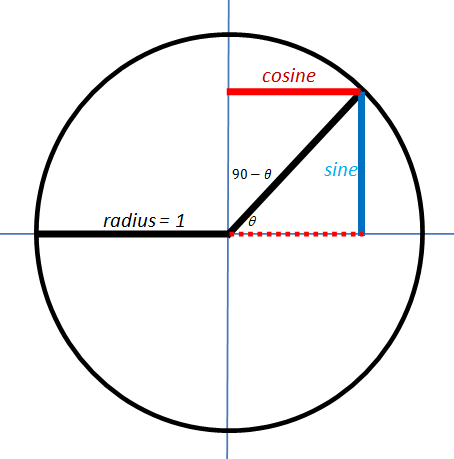We can call that red segment the “bowstring of the complement”, or the “complement’s sine.”  Or we could just call it the cosine!

Now we are going to add two segments to the diagram: one that just touches the circle and one that crosses the circle.  We will use the same names we used for these back in geometry class.  As you can see from the labels, the orange one is called the tangent and the green one is the secant.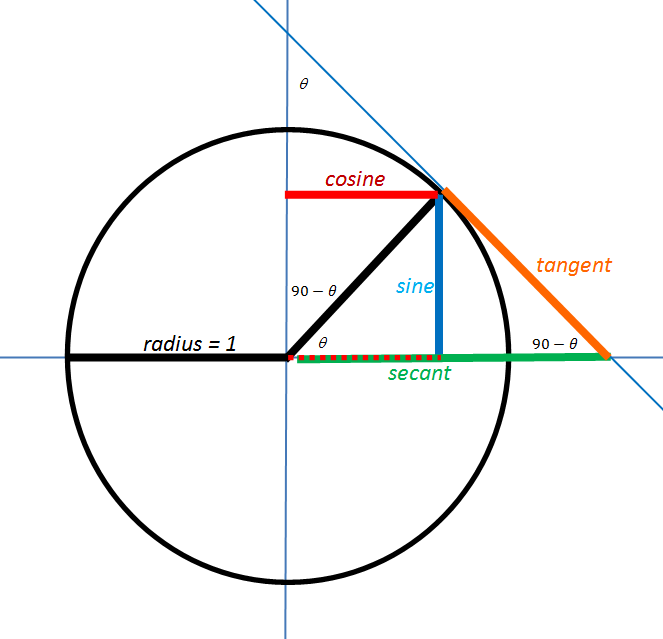Now, as we examine this diagram, we can find a number of interesting pairs of similar triangles.  To discuss them, it would be helpful if we label some points of interest: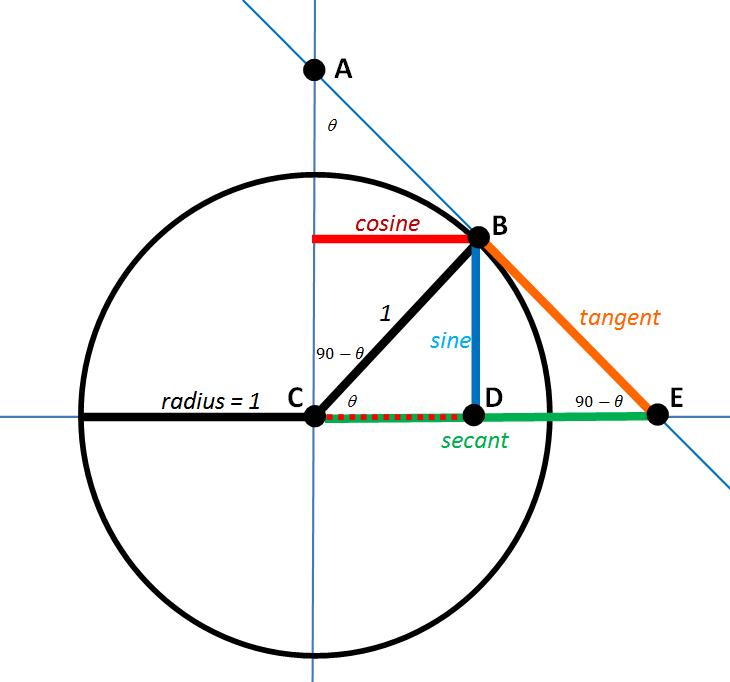Now we have arrived at the key step.

Take a moment to convince yourself that ΔEBC is similar to ΔBDC.

Then, since similar triangles have proportional sides, it must also be true that: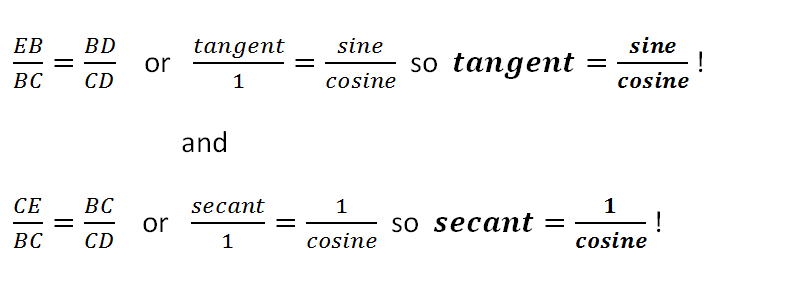These are NOT arbitrarily chosen definitions.  They follow by necessity from the geometric definitions of these terms.

I will pause here to allow everyone to catch their breath.  In the next post, we will finish developing the diagram and add in the Pythagorean identities.